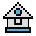Home

# RANDOMIZE Statement

#### Purpose:

To reseed the random number generator.

#### Syntax:

```RANDOMIZE [expression]
RANDOMIZE TIMER```

If expression is omitted, GW-BASIC suspends program execution and asks for a value by displaying the following line:

`Random number seed (-32768 to 32767)?`

If the random number generator is not reseeded, the RND function returns the same sequence of random numbers each time the program is run.

To change the sequence of random numbers every time the program is run, place a RANDOMIZE statement at the beginning of the program, and change the argument with each run (see RND function).

RANDOMIZE with no arguments will prompt you for a new seed. RANDOMIZE [expression] will not force floating-point values to integer. expression may be any numeric formula.

To get a new random seed without prompting, use the new numeric TIMER function as follows:

`RANDOMIZE TIMER `

#### Example 1:

The internal clock can be set at intervals.

```10 RANDOMIZE TIMER
20 FOR I=1 to 5
30 PRINT RND;
40 NEXT I
RUN
.88598 .484668 .586328 .119426 .709225
RUN
.803506 .162462 .929364 .292443 .322921```

#### Example 2:

The internal clock can be used for random number seed.

```5 N=VAL(MID\$(TIME\$, 7, 2)) 'get seconds for seed
10 RANDOMIZE N             'install number
20 PRINT N                 'print seconds
30 PRINT RND               'print random number generated
RUN
36
.2466638
RUN
37
.6530511
RUN
38
5.943847E+02
RUN
40
.8722131```